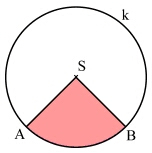Circle segment

A quarter circle with radius 4 has the same content as a circle segment with radius 3. What is the magnitude of the center angle of the circle segment?

α =  160 °

Step-by-step explanation:Did you find an error or inaccuracy? Feel free to write us. Thank you!Tips to related online calculators
Do you have a linear equation or system of equations and looking for its solution? Or do you have a quadratic equation?

You need to know the following knowledge to solve this word math problem:

We encourage you to watch this tutorial video on this math problem: